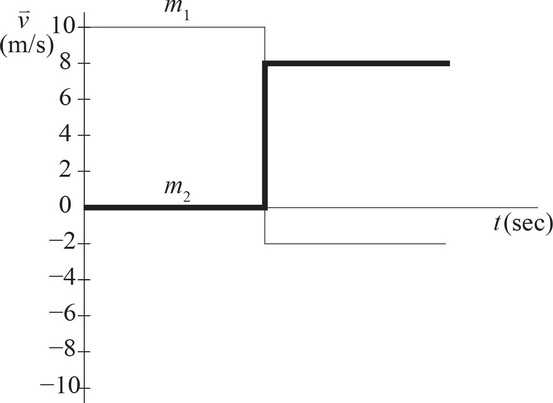# AP Physics 1 Question 318: Answer and Explanation

### Test Information

Question: 3186. Question below refers to the graph above.

Two objects of masses m1 and m2 undergo a collision. The graph above shows their velocities with respect to time both before and after the collision. If m1 = 10 kg, then m2 must be

• A. 5 kg
• B. 10 kg
• C. 15 kg
• D. 20 kg